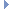neighbours.hpp File Reference

Header file for neighbour list generation. More...

#include <generic.hpp>
#include <mol_sys.hpp>Include dependency graph for neighbours.hpp:This graph shows which files directly or indirectly include this file:

Go to the source code of this file.

## Namespaces

nneigh
Functions for building neighbour lists. This namespace contains functions that build neighbour lists (using brute-force), saving either the atom IDs or atom indices (according to a PointCloud) in a row-ordered vector of vectors. Whether the atom IDs or atom indices (i.e. the indices of the elements in the vector pts inside the PointCloud) are saved, the neighbour lists are constructed such that the first element is the 'central atom', whose neighbours are being saved on that particular line. The central atom is followed by the atom IDs or indices of the nearest neighbours.

## Functions

std::vector< std::vector< int > > nneigh::neighList (double rcutoff, molSys::PointCloud< molSys::Point< double >, double > *yCloud, int typeI, int typeJ)
All these functions use atom IDs and not indices. More...

std::vector< std::vector< int > > nneigh::neighListO (double rcutoff, molSys::PointCloud< molSys::Point< double >, double > *yCloud, int typeI)

std::vector< std::vector< int > > nneigh::halfNeighList (double rcutoff, molSys::PointCloud< molSys::Point< double >, double > *yCloud, int typeI=1)

std::vector< std::vector< int > > nneigh::neighbourListByIndex (molSys::PointCloud< molSys::Point< double >, double > *yCloud, std::vector< std::vector< int >> nList)

std::vector< std::vector< int > > nneigh::getNewNeighbourListByIndex (molSys::PointCloud< molSys::Point< double >, double > *yCloud, double cutoff)

int nneigh::clearNeighbourList (std::vector< std::vector< int >> &nList)
Erases memory for a vector of vectors for the neighbour list. More...

## Detailed Description

Header file for neighbour list generation.

Definition in file neighbours.hpp.